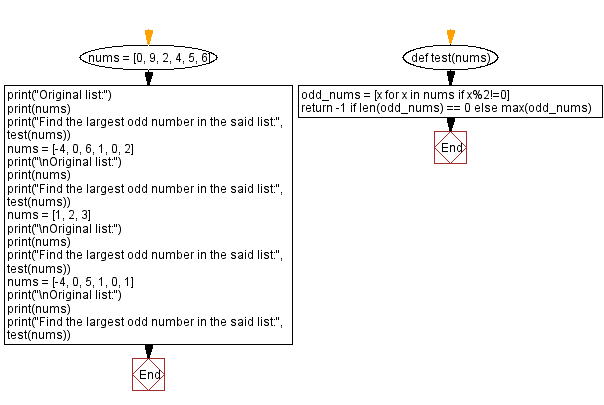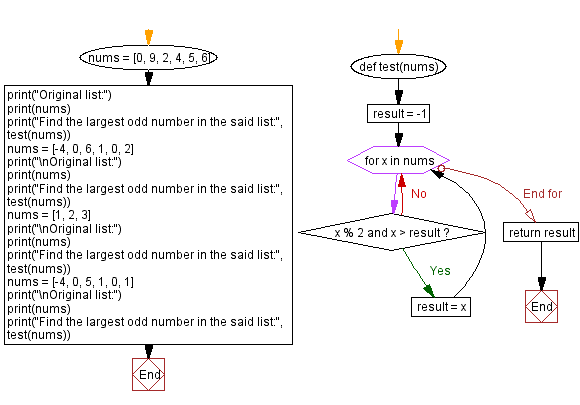﻿ Python - Find the largest odd number in a list of integers# Python Exercises: Find the largest odd number in a list of integers

## Python List: Exercise - 276 with Solution

Write a Python program to find the largest odd number in a given list of integers.

Sample Data:
([0, 9, 2, 4, 5, 6]) -> 9
([-4, 0, 6, 1, 0, 2]) -> 1
([1, 2, 3]) -> 3
([-4, 0, 5, 1, 0, 1]) -> 5

Sample Solution-1:

Python Code:

``````def test(nums):
odd_nums = [x for x in nums if x%2!=0]
return -1 if len(odd_nums) == 0 else max(odd_nums)
nums = [0, 9, 2, 4, 5, 6]
print("Original list:")
print(nums)
print("Find the largest odd number in the said list:", test(nums))
nums = [-4, 0, 6, 1, 0, 2]
print("\nOriginal list:")
print(nums)
print("Find the largest odd number in the said list:", test(nums))
nums = [1, 2, 3]
print("\nOriginal list:")
print(nums)
print("Find the largest odd number in the said list:", test(nums))
nums = [-4, 0, 5, 1, 0, 1]
print("\nOriginal list:")
print(nums)
print("Find the largest odd number in the said list:", test(nums))
```
```

Sample Output:

```Original list:
[0, 9, 2, 4, 5, 6]
Find the largest odd number in the said list: 9

Original list:
[-4, 0, 6, 1, 0, 2]
Find the largest odd number in the said list: 1

Original list:
[1, 2, 3]
Find the largest odd number in the said list: 3

Original list:
[-4, 0, 5, 1, 0, 1]
Find the largest odd number in the said list: 5
```

Flowchart:## Visualize Python code execution:

The following tool visualize what the computer is doing step-by-step as it executes the said program:

Sample Solution-2:

Python Code:

``````def test(nums):
result = -1
for x in nums:
if x % 2 and x > result:
result = x
return result
nums = [0, 9, 2, 4, 5, 6]
print("Original list:")
print(nums)
print("Find the largest odd number in the said list:", test(nums))
nums = [-4, 0, 6, 1, 0, 2]
print("\nOriginal list:")
print(nums)
print("Find the largest odd number in the said list:", test(nums))
nums = [1, 2, 3]
print("\nOriginal list:")
print(nums)
print("Find the largest odd number in the said list:", test(nums))
nums = [-4, 0, 5, 1, 0, 1]
print("\nOriginal list:")
print(nums)
print("Find the largest odd number in the said list:", test(nums))
```
```

Sample Output:

```Original list:
[0, 9, 2, 4, 5, 6]
Find the largest odd number in the said list: 9

Original list:
[-4, 0, 6, 1, 0, 2]
Find the largest odd number in the said list: 1

Original list:
[1, 2, 3]
Find the largest odd number in the said list: 3

Original list:
[-4, 0, 5, 1, 0, 1]
Find the largest odd number in the said list: 5
```

Flowchart:## Visualize Python code execution:

The following tool visualize what the computer is doing step-by-step as it executes the said program:

Python Code Editor:

Have another way to solve this solution? Contribute your code (and comments) through Disqus.

Previous Python Exercise: Sum of all list elements except current element.
Next Python Exercise: Largest, lowest gap between sorted values of a list.

What is the difficulty level of this exercise?

Test your Programming skills with w3resource's quiz.

﻿

## Python: Tips of the Day

Getting sorted iterable (can sort by "compare" function):

```>>> a = [1, 2, -3]
>>> sorted(a)
[-3, 1, 2]

>>> sorted(a,key=abs)
[1, 2, -3]
```